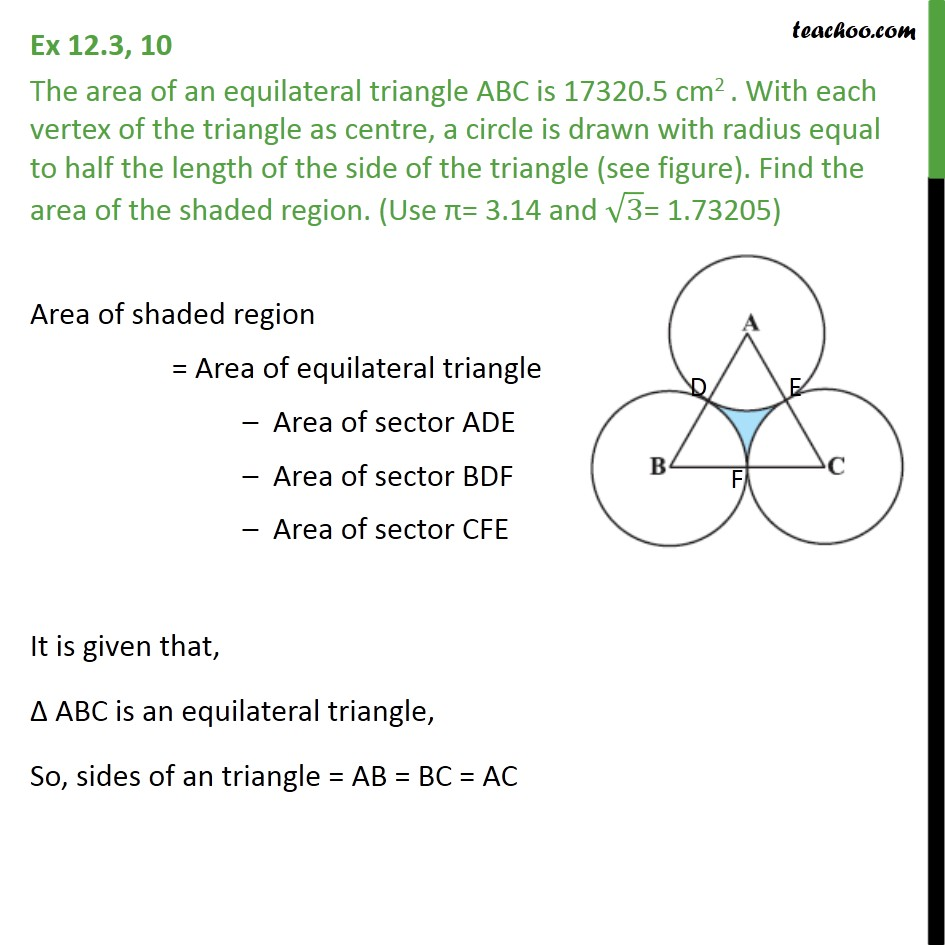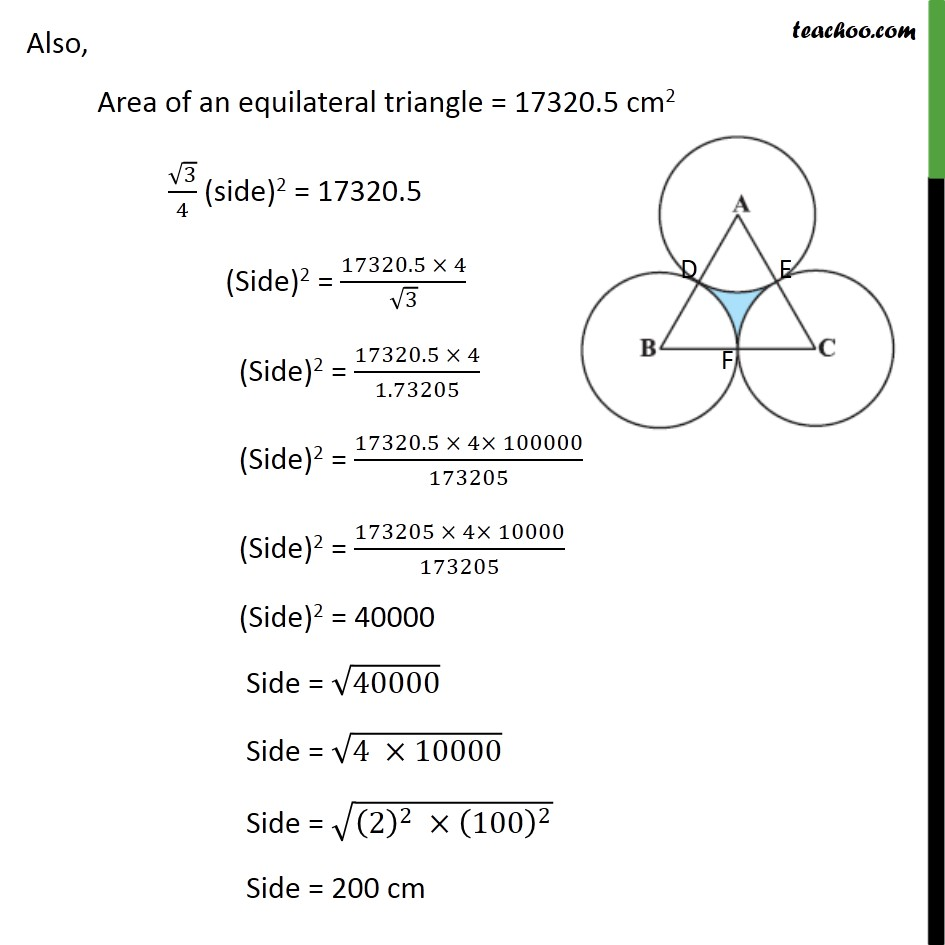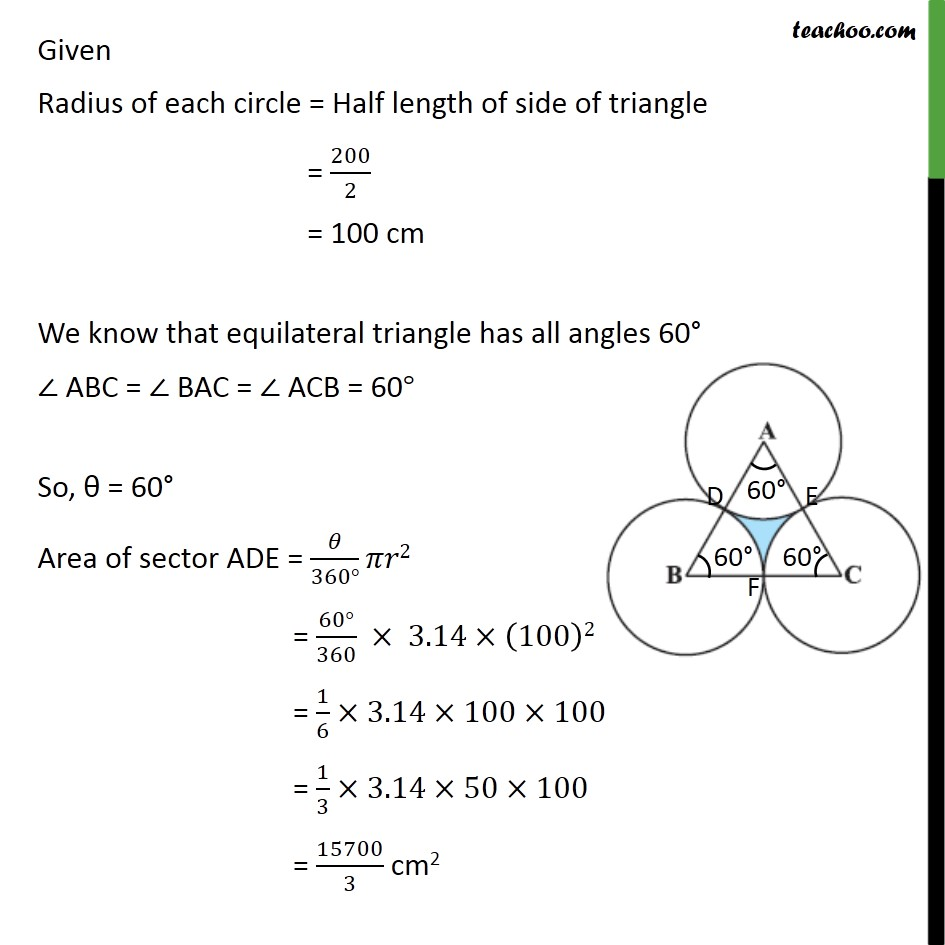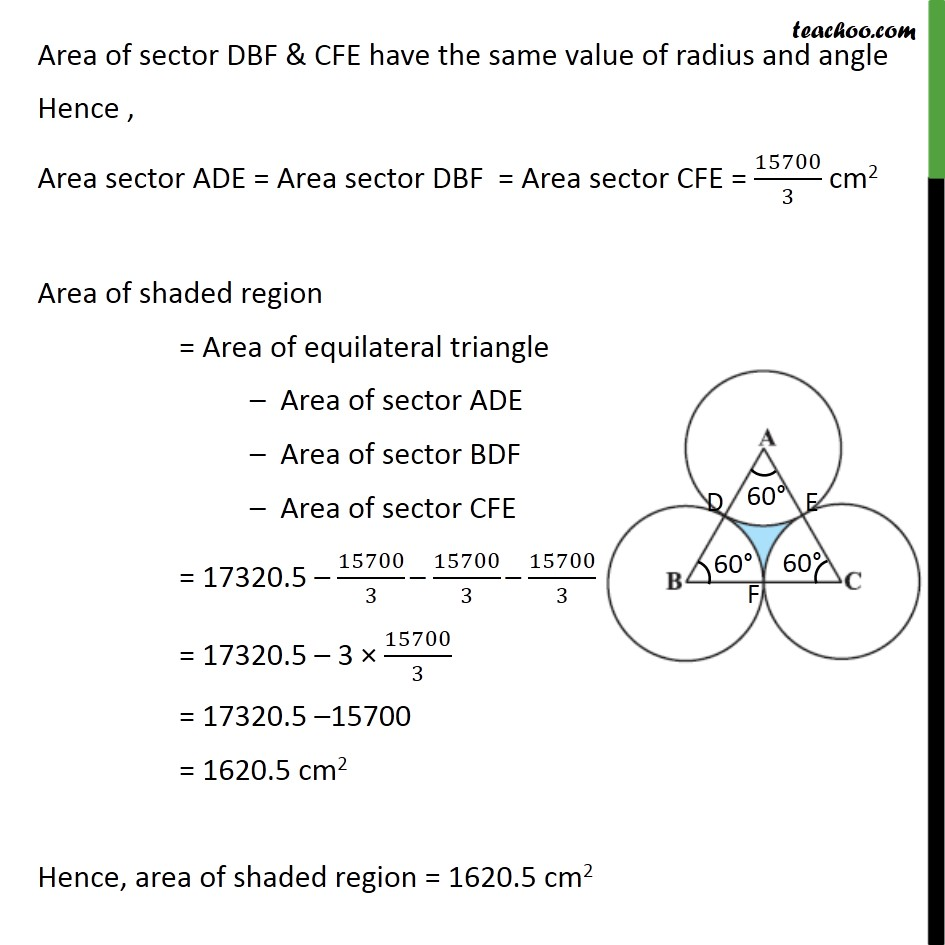1. Chapter 12 Class 10 Areas related to Circles (Term 1)
2. Serial order wise
3. Ex 12.3

Transcript

Ex 12.3, 10 The area of an equilateral triangle ABC is 17320.5 cm2 . With each vertex of the triangle as centre, a circle is drawn with radius equal to half the length of the side of the triangle (see figure). Find the area of the shaded region. (Use = 3.14 and 3= 1.73205) Area of shaded region = Area of equilateral triangle Area of sector ADE Area of sector BDF Area of sector CFE It is given that, ABC is an equilateral triangle, So, sides of an triangle = AB = BC = AC Also, Area of an equilateral triangle = 17320.5 cm2 3/4 (side)2 = 17320.5 (Side)2 = (17320.5 4)/ 3 (Side)2 = (17320.5 4)/1.73205 (Side)2 = (17320.5 4 100000)/173205 (Side)2 = (173205 4 10000)/173205 (Side)2 = 40000 Side = 40000 Side = (4 10000) Side = ((2)^2 (100)^2 ) Side = 200 cm Given Radius of each circle = Half length of side of triangle = 200/2 = 100 cm We know that equilateral triangle has all angles 60 ABC = BAC = ACB = 60 So, = 60 Area of sector ADE = /(360 ) 2 = (60 )/360 3.14 (100)2 = 1/6 3.14 100 100 = 1/3 3.14 50 100 = 15700/3 cm2 Area of sector DBF & CFE have the same value of radius and angle Hence , Area sector ADE = Area sector DBF = Area sector CFE = 15700/3 cm2 Area of shaded region = Area of equilateral triangle Area of sector ADE Area of sector BDF Area of sector CFE = 17320.5 15700/3 15700/3 15700/3 = 17320.5 3 15700/3 = 17320.5 15700 = 1620.5 cm2 Hence, area of shaded region = 1620.5 cm2